Resources: Building polyhedra with Zome

Zome System is a wonderful tool to build polyhedra.

Golden SectionFrom Euclid's definition of the division of a segment into its extreme and mean ratio we introduce a property of golden rectangles and we deduce the equation and the value of the golden ratio.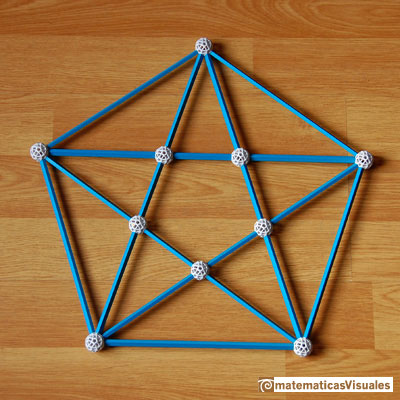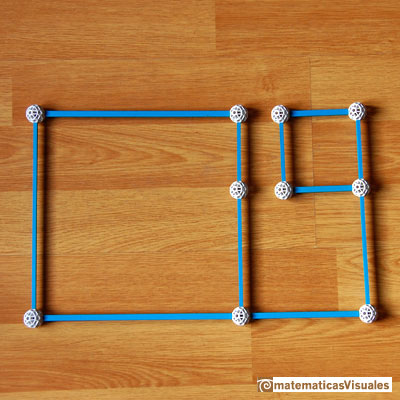Octahedron and Icosahedron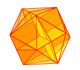The twelve vertices of an icosahedron lie in three golden rectangles. Then we can calculate the volume of an icosahedron

Icosahedron and octahedron, one inside each other, with Zome: .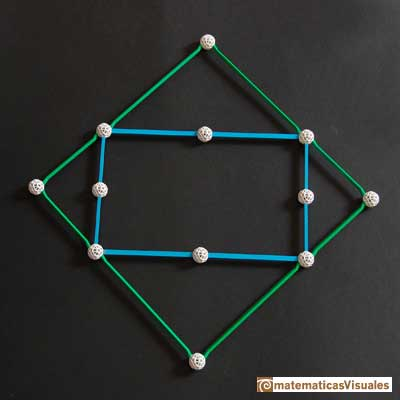DodecahedronSome properties of this platonic solid and how it is related to the golden ratio. Constructing dodecahedra using different techniques.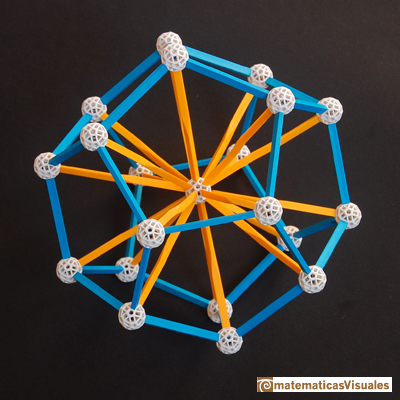Dodecahedron and Icosahedron are dual polyhedra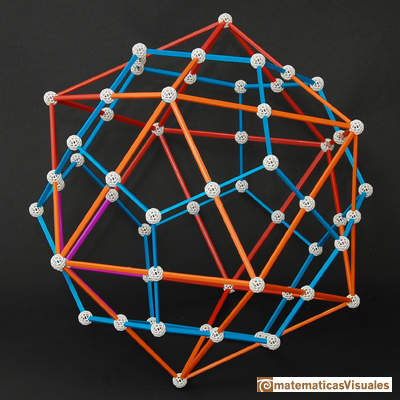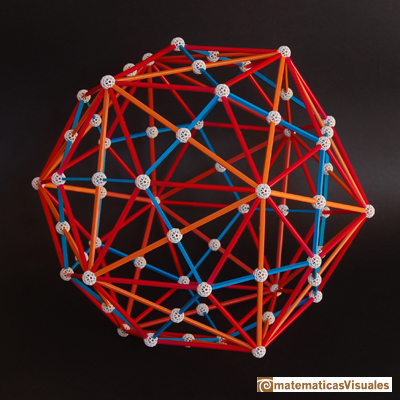Five tetrahedra inside a dodecahedron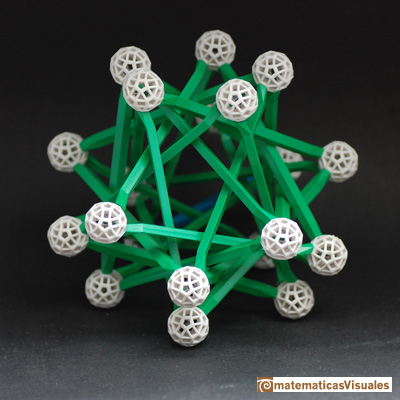Cube inside a dodecahedron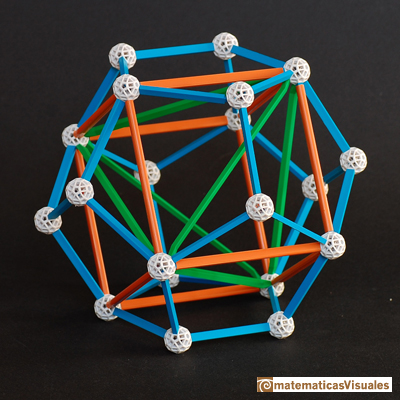Dodecahedron volumeOne eighth of a regular dodecahedon of edge 2 has the same volume as a dodecahedron of edge 1.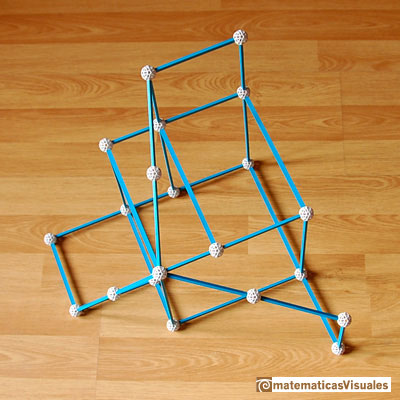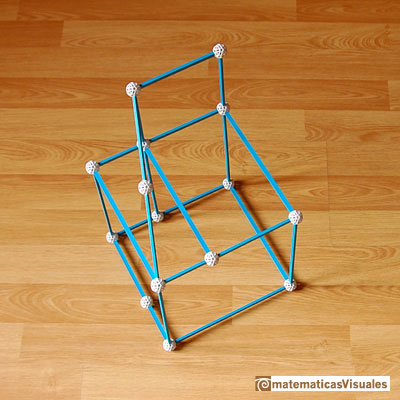CuboctahedronA cuboctahedron is an Archimedean solid. It can be seen as made by cutting off the corners of a cube.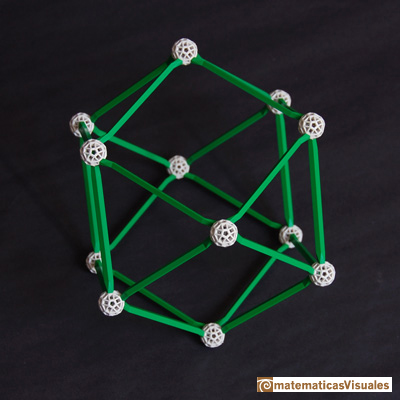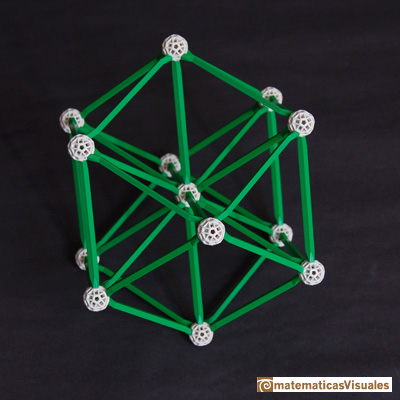More polyhedra with Zome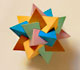Using cardboard you can build beautiful polyhedra cutting polygons and glue them toghether. This is a very simple and effective technique. You can download several templates. Then print, cut and glue: very easy!Simple technique to build polyhedra gluing discs made of cardboard or paper.Italian designer Bruno Munari conceived 'Acona Biconbi' as a work of sculpture. It is also a beautiful game to play with colors and shapes.Material for a session about polyhedra (Zaragoza, 9th May 2014). Simple techniques to build polyhedra like the tetrahedron, octahedron, the cuboctahedron and the rhombic dodecahedron. We can build a box that is a rhombic dodecahedron.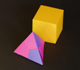Material for a session about polyhedra (Zaragoza, 7th November 2014). We study the octahedron and the tetrahedron and their volumes. The truncated octahedron helps us to this task. We build a cubic box with cardboard and an origami tetrahedron.Material for a session about polyhedra (Zaragoza, 23rd Octuber 2015) . Building a cube with cardboard and an origami octahedron.Material for a session about polyhedra (Zaragoza, 21st October 2016). Instructions to build several geometric bodies.Microarquitectura is a construction game developed by Sara San Gregorio. You can play and build a lot of structures modelled on polyhedra.The volume of a tetrahedron is one third of the prism that contains it.The first drawing of a plane net of a regular tetrahedron was published by Dürer in his book 'Underweysung der Messung' ('Four Books of Measurement'), published in 1525 .The volume of an octahedron is four times the volume of a tetrahedron. It is easy to calculate and then we can get the volume of a tetrahedron.We can cut in half a cube by a plane and get a section that is a regular hexagon. Using eight of this pieces we can made a truncated octahedron.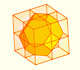Using eight half cubes we can make a truncated octahedron. The cube tesselate the space an so do the truncated octahedron. We can calculate the volume of a truncated octahedron.Leonardo da Vinci made several drawings of polyhedra for Luca Pacioli's book 'De divina proportione'. Here we can see an adaptation of the truncated octahedron.The truncated octahedron is an Archimedean solid. It has 8 regular hexagonal faces and 6 square faces. Its volume can be calculated knowing the volume of an octahedron.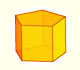We study different prisms and we can see how they develop into a plane net. Then we explain how to calculate the lateral surface area.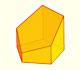Plane nets of prisms with a regular base with different side number cut by an oblique plane.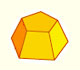Plane net of pyramids and pyramidal frustrum. How to calculate the lateral surface area.